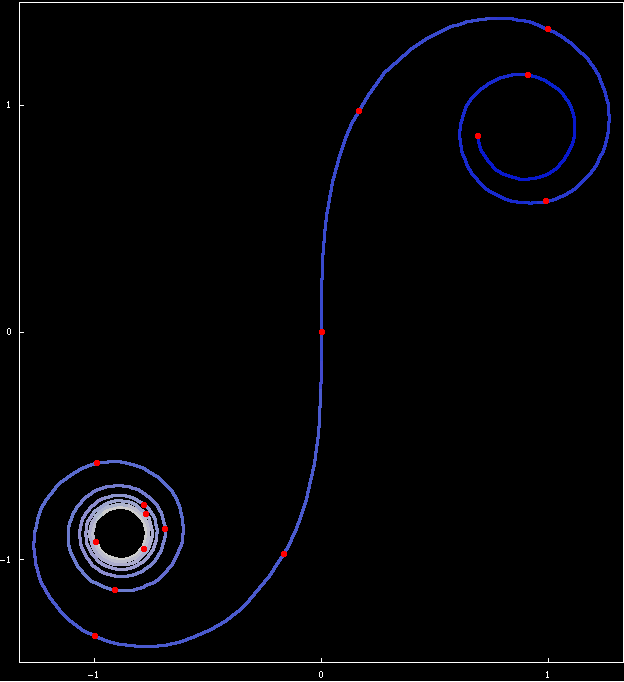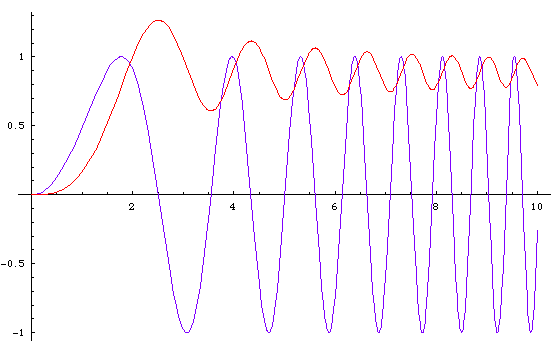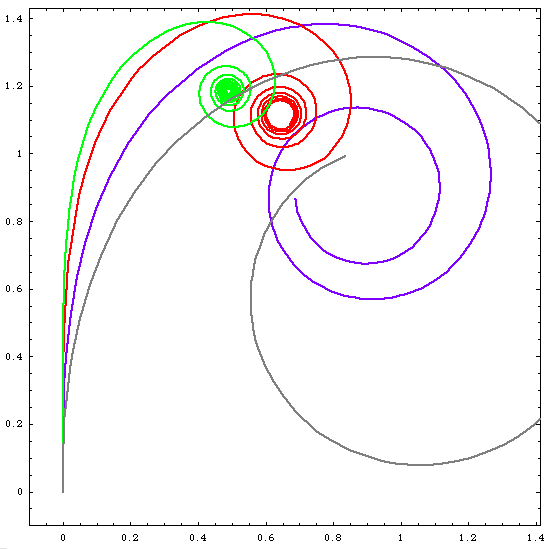# Cornu SpiralThe Cornu Spiral. The segments between each red dots have lengths 1. The curve spirals inwards towards ±{Sqrt[π]/2, Sqrt[π]/2}.

## History

These were considered by Euler at least as early as 1743 in a problem of his celebrated work on the calculus of variations: Methodus inveniendi lineas curvas maxime minimire proprietate gaudentes.

The spiral is also known as Euler Spiral, named after Leonhard Euler, or Cornu Spiral, after Marie Alfred Cornu .

Steven Schwartzman, in The Words of Mathematics Buy at amazon, suggests it derives from Greek kloth (“to twist by spinning”). He suggests that the curve resembles a spinning wheel, and clothoid might be an allusion to Greek goddess Clotho who spun a thread of destiny.

## Description

The clothoid is defined as the curve whose curvature is equal to its length. That is, the further the curve, the more curved it is. Such a curve is necessarily a spiral.

It is a curve invented in the era of differential geometry (in the 1700s), where mathematicians made precise the notion of curvature as the rate the curve changes directions (or, a measure of how “bent” a curve is at a given point on the curve). A theorem called the Fundamental theorem of curves is that a plane curve is essentially defined by its curvature.

## Formula

The clothoid is defined by this parametric formula:

```{ Integrate[ Sin[(x^2)/2], {x,0,t}],Integrate[ Cos[(x^2)/2], {x,0,t}] }
```

## Properties

### Arclength and Curvature Equality

The function to compute the lenth of a curve {xf[t],yf[t]} from a to b is:

`Integrate[ Sqrt[ xf'[t]^2 + yf'[t]^2] , {t,a,b} ]`

Apply this to the clothoid parametric formula above, we obtain t. This means the parametric formula for clothid given above is such that the curve's arclength function is just t itself.

The function to compute the curvature of a curve {xf[t],yf[t]} at b is:

```( -yf'[b] * xf''[b] + xf'[b]*yf''[b]) / (xf'[b]^2 + yf'[b]^2)^(3/2)
```

Apply this to the clothoid parametric formula above, we obtain b. Since the arclength is also t, this means that the clothoid is the curve whose curvature varies identically in value with its length.The blue curve is Sin[x^2/2], the red is Integrate[Sin[m^2/2],{m,0,x}]. The red curve is the area of the blue curve. From the red curve, we see that the value vacillates, and has a limit of “Sqrt[π]/2” (≈ 0.88622).

### Generalization

We can generalized the Cornu Spiral by defining a curve such that, if its arclength is t, then its curvature is t^n. The formula for such a curve is this:

```{ Integrate[ Sin[(x^(n+1))/(n+1)], {x,0,t}],
Integrate[ Cos[(x^(n+1))/(n+1)], {x,0,t}] }
```Three generalized Cornu Spirals. The blue has curvature t^1, red has t^2, green has t^3. The gray has curvature “t^0.5”. For all curves, the parameter t goes from 0 to 5.

When n==0, the curve becomes a circle, with curvature t^0, as expected.

Cornu Spirals

clothoid_n.nb